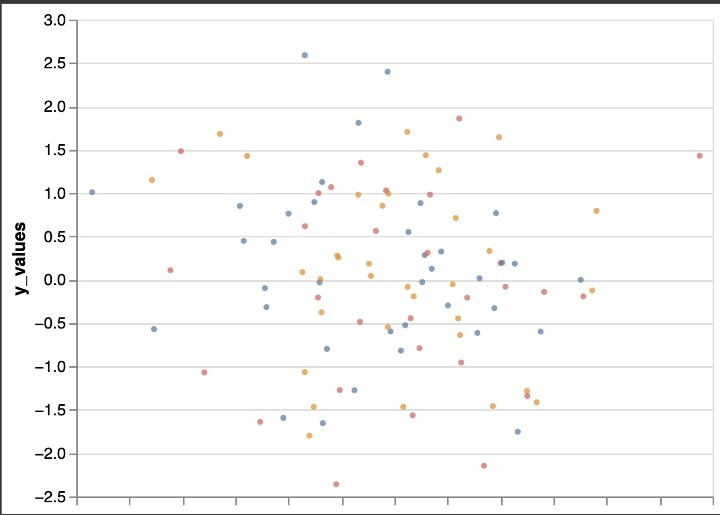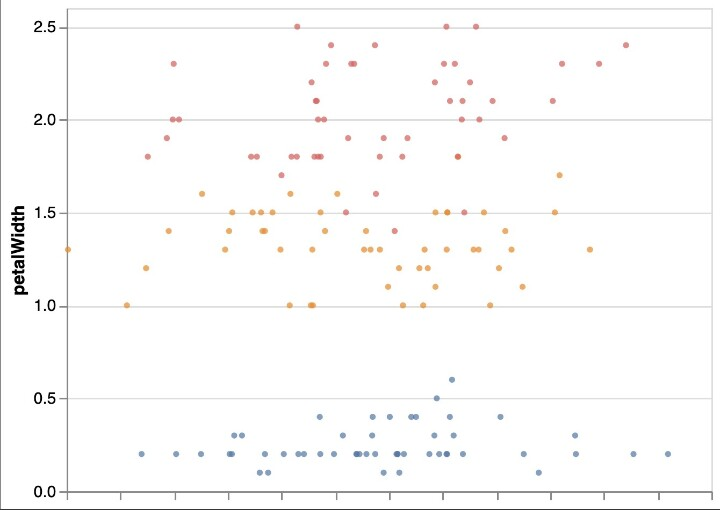# How to Make Stripplot with Jitter in Altair Python?

This tutorial will explain how to make Stripplot with Jitter in Altair Python. It is quick and easy to visualize a dataset containing a continuous and a categorical variable using a strip plot with jitter in Altair Python. In a strip plot, one of the variables is categorical, and the other is continuous. Strip plots are a sort of scatter plot. We can see the distribution of the continuous variable for each category by looking at the data points as individual points along the categorical axis. Spreading out the data points on the plot with jitter makes it simpler to examine how the data are distributed.

Using the mark_circle() function to construct the plot and the jitter() function to add the jitter effect, we can create a strip plot with jitter in Altair Python. The encoding argument must first be used to specify the x and y variables for the plot. The plot is then made using the mark_circle() function, and the jitter() function is used to add the jitter effect. The plot can also be modified by altering the axis labels, the color scheme and adding a title. We may make a readable and instructive strip plot with jitter in Altair Python by following these straightforward methods.

## Syntax

Altair is a Python library that can be used to create strip plots with jitter. Here is an example of the syntax for creating a strip plot with jitter using Altair −

import altair as alt

# create a stripplot with jitter using Altair
alt.Chart(df).mark_circle(size = 14).encode(
x = alt.X('jitter:Q', title = None, axis = alt.Axis(ticks = True, grid = False, labels = False), scale = alt.Scale()),
y = alt.Y('Y:Q', scale = alt.Scale()),
color = alt.Color('C:N', legend = None),
).transform_calculate(
Jitter = 'sqrt(-2*log(rand()))*cos(2*PI*rand())',
)


The given code creates a stripplot with jitter using Altair. The transform_calculate() method generates a Gaussian jitter for the x-axis by calculating the square root of the negative two times the natural logarithm of a random number generated using the rand() method in Python's random module and then multiplying it by the cosine of two times pi times another random number generated using the same rand() method. This generates a jitter value added to the 'jitter' column in the DataFrame. This jitter value is then mapped to the x-axis using the 'jitter:Q' encoding in the alt.X() method.

## Example

The below code generates a stripplot with jitter using the Altair visualization library in Python. The code first creates a custom dataset using pandas.DataFrame()contains 100 randomly generated x-values, y-values, and categories. The x and y values represent the coordinates of the points in the plot, while the category column determines the color of each point.

The alt.Chart() function is then used to create a chart object, and the mark_circle() function is called to specify that the plot should be a circle. The encode() method is used to specify how the data should be mapped to the plot's visual properties, such as the x and y positions and the point color. In this case, the x encoding uses a calculated field called jitter to add jitter to the x-axis, while the y encoding specifies the y-values. The color encoding uses the category column to color the points, and the legend=None argument removes the legend. Finally, the transform_calculate() function is used to calculate the jitter field using a formula based on a random number generator, which adds a small amount of random noise to the x-values and spreads the points out horizontally.

import altair as alt
import pandas as pd
import numpy as np

# create a custom dataset
custom_data = pd.DataFrame({
'x_values': np.random.randn(100),
'y_values': np.random.randn(100),
'category': np.random.choice(['A', 'B', 'C'], 100)
})

# create a stripplot with jitter using Altair
alt.Chart(custom_data).mark_circle(size = 14).encode(
x=alt.X('jitter:Q', title = None,
axis = alt.Axis(ticks = True, grid = False, labels = False), scale = alt.Scale()),
y=alt.Y('y_values:Q', scale=alt.Scale()),
color=alt.Color('category:N', legend = None),
).transform_calculate(
jitter='sqrt(-2*log(random()))*cos(2*PI*random())',
)


## Output## Example

This example shows the creation of a stripplot with jitter in Altair using the Iris dataset. The code first imports the necessary libraries, including Altair and the Iris dataset, from the vega_datasets library. It then creates an Altair chart using the mark_circle method to create a circle for each data point and encodes the x, y, and color variables using the Altair X, Y, and Color classes, respectively.

This example shows the creation of a stripplot with jitter in Altair using the Iris dataset. The code first imports the necessary libraries, including Altair and the Iris dataset, from the vega_datasets library. It then creates an Altair chart using the mark_circle method to create a circle for each data point and encodes the x, y, and color variables using the Altair X, Y, and Color classes, respectively.

import altair as alt
from vega_datasets import data

iris = data.iris()

# create a stripplot with jitter using Altair
alt.Chart(iris).mark_circle(size = 14).encode(
x = alt.X('jitter:Q', title = None, axis = alt.Axis(ticks = True, grid = False, labels = False), scale = alt.Scale()),
y = alt.Y('petalWidth:Q', scale = alt.Scale()),
color = alt.Color('species:N', legend = None),
).transform_calculate(
jitter = 'sqrt(-2*log(random()))*cos(2*PI*random())',
)


## Output## Conclusion

In conclusion, using jitter to create a stripplot is useful for displaying the distribution of data points and their variability. The Python Altair package makes it simple and effective to complete this operation. Users can make an instructive and aesthetically pleasing plot by following the instructions provided in this article, which include importing the required libraries, loading the data, and encoding the x, y, and color variables. The plot is further improved by the inclusion of jitter using the transform_calculate method since it is now simpler to identify specific data points and patterns within the data.

Overall, Altair is a strong Python data visualization tool, and using jitter to create stripplots is just one illustration of its capability. You may make a variety of powerful and educational visualizations by experimenting with various datasets and visual encodings. The possibilities for data visualization are unlimited, thanks to Altair's simple syntax and robust capabilities.

Updated on: 12-May-2023

168 Views